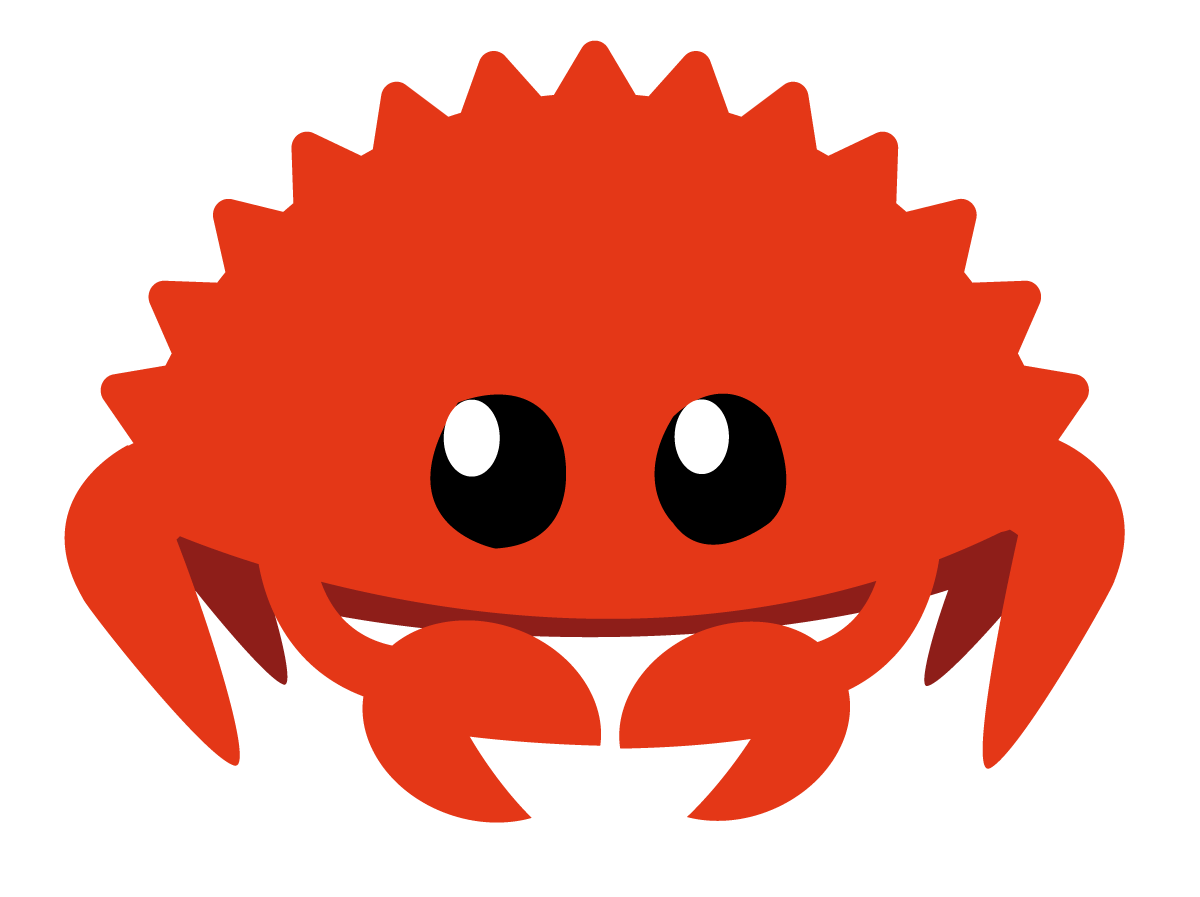# Rust - Vector

eslerkang·2022년 3월 10일
0

## Rust

목록 보기
3/10## Vector

Vector(vec) of rust is similar to Array.
But it is more easy to push or pop. Also when we talk about type, length of vector is negligible. So Array's type is different by length, but Vector's type only decided by it's components type(Vec<String>).
We call the vector's type as Vec<T>, and T is generic that means 'some type'.
We can check the capacity of vector by .capacity()

fn main() {
let name1 = String::from("Kang");
let name2 = String::from("Kim");

let mut vec = Vec::new();
println!("Space for my vec: {}", vec.capacity());
vec.push(name1);
println!("Space for my vec: {}", vec.capacity());
vec.push(name2);
// let vec = vec![name1, name2];
println!("Space for my vec: {}", vec.capacity());
println!("{:?}", vec);
}

To make new vector, we can use Vec::new() or Vec::from(). Also, we can use macro vec![]. Its same as making array, like vec![name1, name2].
As it's type is decided by T(generic), we cannot make a vector empty. We can declare the vector as empty, but we have to assign it's type or at least one component.